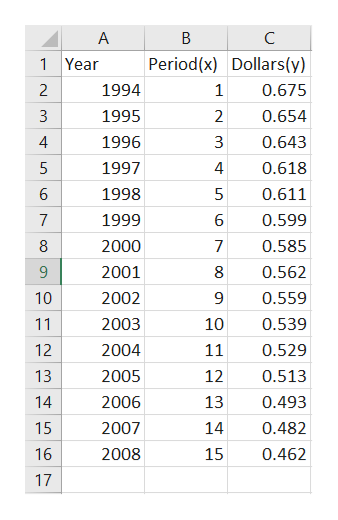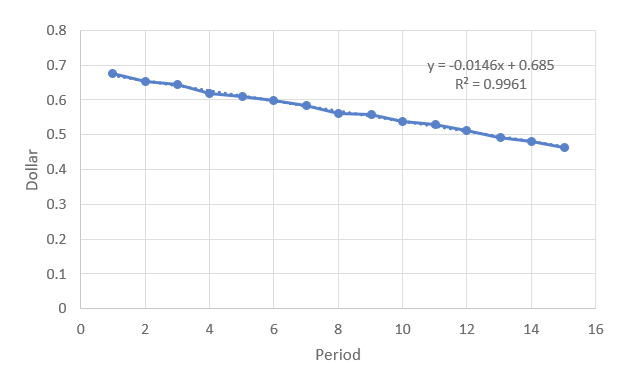# The value y (in 1982–1984 dollars) of each dollar paid by consumers in each of the years from 1994 through 2008 in a country is represented by the ordered pairs.(1994, 0.675)        (1995, 0.654)(1996, 0.643)        (1997, 0.618)(1998, 0.611)        (1999, 0.599)(2000, 0.585)        (2001, 0.562)(2002, 0.559)        (2003, 0.539)(2004, 0.529)        (2005, 0.513)(2006, 0.493)        (2007, 0.482)(2008, 0.462)        (b) Use the regression feature of the spreadsheet software program to find a linear model for the data. (Let trepresent time. Round your numerical values to four decimal places.)y = (c) Use the model to predict the value (in 1982–1984 dollars) of 1 dollar paid by consumers in 2010 and in 2011. (Round your answers to two decimal places.)

Question
374 views

The value y (in 1982–1984 dollars) of each dollar paid by consumers in each of the years from 1994 through 2008 in a country is represented by the ordered pairs.

 (1994, 0.675) (1995, 0.654) (1996, 0.643) (1997, 0.618) (1998, 0.611) (1999, 0.599) (2000, 0.585) (2001, 0.562) (2002, 0.559) (2003, 0.539) (2004, 0.529) (2005, 0.513) (2006, 0.493) (2007, 0.482) (2008, 0.462)

(b) Use the regression feature of the spreadsheet software program to find a linear model for the data. (Let trepresent time. Round your numerical values to four decimal places.)
y =

(c) Use the model to predict the value (in 1982–1984 dollars) of 1 dollar paid by consumers in 2010 and in 2011. (Round your answers to two decimal places.)

check_circle

Step 1

It is a time series data, so we convert the year in periods
Entering the data in excel as shown belowhelp_outlineImage TranscriptioncloseA B C 1 Year Period(x) Dollars(y) 2 1994 1 0.675 0.654 3 1995 2 4 1996 3 0.643 1997 4 0.618 6 1998 0.611 0.599 7 1999 8 2000 7 0.585 9 2001 8 0.562 10 2002 0.559 11 2003 10 0.539 2004 12 11 0.529 13 2005 12 0.513 13 14 2006 0.493 15 2007 14 0.482 16 2008 15 0.462 17 LC LC OC fullscreen
Step 2

b) Finding the equation of least square in excel.
It can be found by plotting the scatter plot in excel.

Steps to plot scatter plot on excel

select the data From B1 to C60 in excel-> Go to insert->Go to scatter diagram-> select scatter diagram with line-> scatter diagram will be plotted.

Equation can be obtained by clicking on + sign on the plot, then on going tredline-> more options-> then select display equation on chart .

Equation on chart will be displayed

The equation obtained is

y = -0.0146x + 0.685help_outlineImage Transcriptionclose0.8 y-0.0146x+0.685 R2 0.9961 0.7 0.6 0.5 0.4 0.3 0.2 0.1 0 0 2 4 6 10 14 16 Period 12 LO et Dollar fullscreen
Step 3

c)

Value of dollar paid by consumers in 2010 and 2011 is
Period corresponding to 2010 is 17
Putting the value of x =17 in least square regression line
y = -0.0146x + 0.685
y = -0.0146 *17 + 0.685= 0.4368
Period correspo...

### Want to see the full answer?

See Solution

#### Want to see this answer and more?

Solutions are written by subject experts who are available 24/7. Questions are typically answered within 1 hour.*

See Solution
*Response times may vary by subject and question.
Tagged in

### Statistics